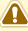Today, I was studying financial formulas in excel. In second formula, I have to learn to calculate DDB. But I did not know to calculate  double declining balance method of depreciation manually. Actually, I had studied 10 methods of calculation of depreciation.

Names of these methods are fixed Instalment method, reducing Instalment method, annuity method, sum of years digits method, depreciation fund method, insurance policy method revaluation method, machine hour rate method mileage method and depletion method. But double declining balance method of depreciation was not in it. So, Before knowing its excel formula, it was very necessary for me to know to calculate depreciation as per this method. After studying some online resources, I am ready to write something on this.

Double declining balance method of depreciation is the mixture of straight line method and diminishing balance method of depreciation. In this method, first of all we calculate the rate of depreciation with using straight line method. After this, we double this rate and calculate first year depreciation. Now, we deduct this depreciation  from initial value of asset and then with double rate, we calculate the second year's depreciation. Now, value will be decline every year and depreciation is calculated on this declined value.

Following example will clear the meaning of double declining balance method of depreciation.

For example, you go to market and buy a machine of Rs. 1,00,000. As per your estimation its active life is 10 years. After this, it can be sold Rs. 1000  in scrap.

1. Now, we use straight line method for calculation of first year depreciation.

= Initial cost / Active life of machine

= 1,00,000 - 1000  / 10 = Rs. 9900

2. Now, convert it in % form.

depreciation / initial cost - scrap  X 100

9900/ 100000 - 1000 X 100 = 10%

3. Now, we double this rate

10 X 2 = 20%

4. Calculate first year depreciation with this rate

1,00,000 X 20% = Rs. 20,000

5. Deduct this depreciation from 100000

100,000 - 20000 = 80,000

6. Calculate the depreciation on 80000 with 20% rate

80,000 X 20% = Rs. 16000

7. Deduct this depreciation from 80000

80000 - 16000 = 64000

Like this, we will calculate depreciation of rest of period.

Importance of Double Declining Balance Method of Depreciation

1. Double declining balance method, we use double of normal rate of depreciation. So, it will be helpful to show more depreciation in the beginning of the year in our income statement. So, it will be helpful to cover the big capital cost from our revenues.

2. Many countries' tax system allow double declining balance method of depreciation as option, so, we can use it for saving tax because depreciation expense will decrease double the value of current earning.

3. With this, company can easily reserve more money for maintaining asset in beginning years of asset.

Important Fact : When we calculate the depreciation with double rate, we need not deduct the scrap value. I was doubt for deducting scrap value because About.com's content has little mistake. It has deducted scrap value but after reading wikipedia content  and calculating double declining balance method of depreciation and calculating depreciation through excel formula, it is sure that double rate will be charged on the initial cost of asset..

## \$type=three\$a=hide\$cm=hide\$s=hide\$show=/2019/06/10-steps-to-become-better-investor.html\$l=hide

Name

false
ltr
item
Accounting Education: Double Declining Balance Method of Depreciation
Double Declining Balance Method of Depreciation
http://4.bp.blogspot.com/-5roGi5fT1nc/T8zIU4JoZ2I/AAAAAAAAH1o/bzVUNsH9XqU/s1600/Copy+of+warning.PNG
http://4.bp.blogspot.com/-5roGi5fT1nc/T8zIU4JoZ2I/AAAAAAAAH1o/bzVUNsH9XqU/s72-c/Copy+of+warning.PNG
Accounting Education
https://www.svtuition.org/2012/06/double-declining-balance-method-of.html
https://www.svtuition.org/
http://www.svtuition.org/
http://www.svtuition.org/2012/06/double-declining-balance-method-of.html
true
2410664366776677676
UTF-8